Painting rooms

If Dano paint three hours daily given work he complete in 7.5 days. How many hours a day would have to work to finish the job 1.5 days earlier?

Result

x =  3.75 h

Solution:

3*7.5 = x*(7.5-1.5)

6x = 22.5

x = 154 = 3.75

Calculated by our simple equation calculator.

Leave us a comment of this math problem and its solution (i.e. if it is still somewhat unclear...):Be the first to comment!To solve this verbal math problem are needed these knowledge from mathematics:

Do you have a linear equation or system of equations and looking for its solution? Or do you have quadratic equation? Most natural application of trigonometry and trigonometric functions is a calculation of the triangles. Common and less common calculations of different types of triangles offers our triangle calculator. Word trigonometry comes from Greek and literally means triangle calculation.

Next similar math problems:

1. Four workers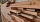Four workers will lay the floor of the gym in six hours. After an hour of working together, one of the workers left to the doctor. How long before the remaining three workers finish their work?
2. NovakNovak needed to dig up three of the same pit in the garden. The first pit dug father alone for 15 hours. His second dig son helped him and it did that in six hours. The third pit dug son himself. How long it took him?
3. PumpsAfter the floods, four equally powerful pumps exhausted water from the flooded cellar in 6 hours. How many hours would take a drained out with three equally powerful pumps?
4. Contract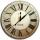8 employees complete the contract for 65 hours. After 17 hours, 3 employees had to leave for another job. How many more hours will the job on contract be fulfilled?
5. Sprayers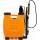The vineyard can be sprayed first sprayer in 5 hours and with the second in 8 hours. How long would it take if we use sprayers both at once?
6. WorkersTwo workers will construct the stage at the festival in 54 hours. Organizers need to shorten the assembly time by 42 hours. How many workers must the assembly company send?
7. PumpsFive pumps pumped 1,800 hl of water for 3 hours. How many hectoliters of water pump same two powerful pumps for six hours?
8. Working together on projectTwo employees working on the project. First make it alone for 20 days the second for 24 days. How many days will take to complete project if 5 days they work together then first will take two days holiday and after returning they completes a project toget
9. Five pumpsThe water tank is filled with two pumps in 48 minutes. How long would it take to fill it with 5 same pumps?
10. Hectoliters of waterThe pool has a total of 126 hectoliters of water. The first pump draws 2.1 liters of water per second. A second pump pumps 3.5 liters of water per second. How long will it take both pumps to drain four-fifths of the water at the same time?
11. Lumberjacks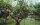Fifteen lumberjacks would take 12 days to destroy the broken trees. How many days would this work require if 5 workers added to it after 4 days?
12. HighwaySix trucks will be used to build the roadside 18 days. After 3 days, two more trucks were sent to build. How long will it take for material move?
13. Six yearsIn six years Jan will be twice as old as he was six years ago. How old is he?
14. Proportion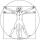write the following proportion 8 is to 64 as to 2 is to x
15. Simple equationSolve for x: 3(x + 2) = x - 18
16. EquationSolve the equation: 1/2-2/8 = 1/10; Write the result as a decimal number.
17. Simply equationSolve this equation for x: ?